HOME plateWON | World!OfNumbers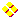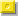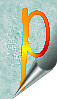Palindromic Primes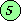Page 5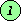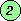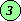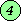Circular PrimesHome PrimesUndulating PrimesSums of PrimesPalindromic Primes

[ April 5, 2021 ]
Palindromic Primes from ninedigitals
by Alexandru Petrescu

We are looking for 19 digits palprimes obtained by concatenating
a ninedigital number with its reversal, but separated by a central
extra digit d.
Example:  145632987 789236541
Obviously d can't be: 0, 3, 6 or 9.

May I present here the table with some statistics and the
lowest/greatest possible solutions.

Central
digit
Number of
Palprimes
The lowest ninedigitalThe greatest ninedigital
14965123458679987653412
13802123459867987654321
14216123456789987653124
15155123456978987654312
14728123456897987654312
14066123456978987654321

[ March 8, 2005 ]
Palindromic primes embedded between two ones
by Zakir Seidov

Take 11 (the only 2-d palprime).

1. Two 1-d palprimes inserted in 11 giving palprimes are
3 and 5.
So 131 and 151 are palprimes.

2. Three 3-d palprimes inserted in 11 giving palprimes are
131, 383, 797.
So 11311, 13831, 17971 are palprimes.

3. Nine 5-d palprimes inserted in 11 giving palprimes are
11411, 16061, 16361,
19391, 33533, 36263,
73037, 75557, 79397.

4. Ninety three 7-d palprimes inserted in 11 giving palprimes are
1074701, 1126211, 1145411, 1175711, 1221221, 1243421, 1287821, 1303031, 1311131, 1328231,
1363631, 1489841, 1579751, 1600061, 1707071, 1748471, 1761671, 1881881, 1884881, 1895981,
1917191, 1936391, 1958591, 1969691, 1988891, 3002003, 3103013, 3155513, 3158513, 3267623,
3368633, 3452543, 3485843, 3586853, 3673763, 3709073, 3717173, 3728273, 3763673, 3769673,
3793973, 3799973, 3804083, 3829283, 3864683, 3913193, 3916193, 3938393, 7041407, 7082807,
7129217, 7134317, 7156517, 7276727, 7300037, 7352537, 7388837, 7434347, 7562657, 7611167,
7666667, 7669667, 7693967, 7865687, 7941497, 7977797, 7985897, 7996997, 9002009, 9043409,
9046409, 9049409, 9109019, 9196919, 9231329, 9289829, 9338339, 9400049, 9439349, 9493949,
9504059, 9586859, 9632369, 9709079, 9711179, 9752579, 9807089, 9845489, 9908099, 9921299,
9932399,9935399,9965699.

5. 421 are 9-digit palprimes (from a total of 5172) which when inserted in 11 give 1pp1 palprimes. See also A088269, A103992.
.

6. Now Q is shifted to 11-d palprimes.

Digits in PPPP_pure1_PP_13_PP_37_PP_79_PP_9
142???
3153???
5939???
766893???
95172421???
1142042????

Everyone with some spare time is invited to complete the above tabel.

Assignment _1_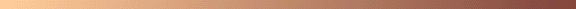Will the following ever happen again ?Is the number 10501 a unique palindromic prime ?
When added together with its preceding prime and its following prime, the result is again a palindromic prime.

10499 + 10501 + 10513 = 31513
Consult also Carlos Rivera's Problems & Puzzles - Puzzle 7 website.

And as a pure coincidence we notice that :

adding the surrounding primes creates another palindrome !
10499 + 10513 = 21012
our palindrome can be expressed as the sum of three consecutive primes !
10501 = 3491 + 3499 + 3511

Jud McCranie gives examples with more than three consecutive primes
that have a palindromic term in the sum somewhere.
787 = 149 + 151 + 157 + 163 + 167
9419149 = 1883759 + 1883773 + 1883857 + 1883879 + 1883881
757 = 97 + 101 + 103 + 107 + 109 + 113 + 127
929 = 83 + 89 + 97 + 101 + 103 + 107 + 109 + 113 + 127
106555601 = 9686791 + 9686819 + 9686837 + 9686839 + 9686851 +
9686867 + 9686869 + 9686879 + 9686921 + 9686951 + 9686977

[ May 15, 2003 ]
Giovanni Resta (email) found eight more solutions to this assignment. Well done!
It pleases me a lot that this old puzzle (five years since its first publication!) still grabs
someone's attention. Giovanni scanned exhaustively all possible solutions
between 10501 and 324456535654423. The list now goes as follows :

10499 + 10501 + 10513 = 31513

324456535654403 + 324456535654423 + 324456535654553 = 973369606963379
326151616151597 + 326151616151623 + 326151616151659 = 978454848454879
332526262625197 + 332526262625233 + 332526262625369 = 997578787875799
11708180908180709 + 11708180908180711 + 11708180908180733 = 35124542724542153
12829181718192793 + 12829181718192821 + 12829181718192869 = 38487545154578483
12907072927070909 + 12907072927070921 + 12907072927070953 = 38721218781212783
12918271817281913 + 12918271817281921 + 12918271817281949 = 38754815451845783
32928090809082919 + 32928090809082923 + 32928090809082947 = 98784272427248789

[ June 10, 2003 ]
Jens Kruse Andersen (email) found many big solutions and could find thousands
of "small" solutions (with 19-39 digits) in a day.

For all the details of his clever approach I refer to Carlos Rivera's webpage at http://www.primepuzzles.net/puzzles/puzz_007.htm.

Here is just one solution (303 digits) from his email that
I prefer above the others because of its palindromic length !

p = 10302 + 10*(7*10301-70)/99 + 10140*22001012200100221010022 + 1
Let w be "0" followed by 69 concatenations of "70".
Then p is the concatenation 1w92708082907170928080729w1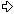Solution equation: (p-138) + (p) + (p+158) = (3p+20)

These 4 primes were proved with Marcel Martin's Primo.

Jens's current record is a solution with 527 digits. Amazing!

```

```

Assignment _2_Can you find larger palindromic primes than

 9918199 3306049 + 3306059 + 3306091

that is the sum of three or more consecutive primes ?

Jud McCranie did !

They were all sent to me by email starting from [ August 26 & September 28, 1998 ].
Note that some palindromic primes have very interesting patterns !
11155555111 - a generalized plateau prime - is expressible as 3 and 7 consecutive primes (see table (!)).

[ June 10, 2003 ]
Jens Kruse Andersen (email) found three titanic solutions (1001 digits).

For the strategy of his clever approach I refer to Carlos Rivera's webpage at http://www.primepuzzles.net/puzzles/puzz_023.htm.

The solutions to assignment 1 are also valid here, but a search designed for
this problem can find bigger solutions.

Here is the second solution of his triplet :

p = 101000 + 1308107018031*10494 + 1Solution equation: (x–3772) + (x–2094) + (x+5868), where x = (p–2)/3

The palprimes were proved with PrimeForm/GW and all the other primes with Marcel Martin's Primo.

Impressive, to say the least, Jens !

```

```

Assignment _3_Try extending one or more of the following seven lists

```

```

Sources Revealed and Related Internet Sites

 Neil Sloane's "Integer Sequences" Encyclopedia can be consulted online : Neil Sloane's Integer Sequences The palindromic primes are already categorised. %N Palindromic primes. under A002385 and %N The number of prime palindromes with n digits. under A016115. Can you grasp and differentiate the following sequences ? %N Palindromic reflectable primes. under A007616. %N Reflectable emirps. under A007628. %N Primes whose reversal is prime. under A007500. %N Emirps (primes whose reversal is a different prime). under A006567. Click here to view some of the author's [P. De Geest] entries to the table. Click here to view some entries to the table about palindromes.

The site The largest known primes keeps monthly updates also about palindromic primes.
Note that the length of this giant is palindromic too (no coincidence!).
The largest one in the list [ dating from April 28, 1999 ] is 10^30802 + 1110111*10^15398 + 1 and is 30803 digits long !
Harvey Dubner published various articles about palindromic primes in the Journal of Recreational Mathematics.
Most of Harvey's record palindromic primes are on display.The Top Ten by Rudolph Ondrejka

In Keith Devlin book All the Math that's Fit to Print chapter 92 we read about the largest known palindromic prime number (back in 1987 !).
It starts and ends with a 1, has a single 5 in the middle, and zeros everywhere else for a total of 2.977 digits.

Albert H. Beiler wrote in his book "Recreations in the Theory of Numbers" Second Edition 1966 page 222 :
Primes can even be palindromic (reading the same backward as forward) and be in arithmetic progression.
Four such sets of four primes are:
(13931, 14741, 15551, 16361), (10301, 13331, 16361, 19391),
(70607, 73637, 76667, 79697), (94049, 94349, 94649, 94949).
The respective common differences are 810; 3030; 3030 and 300.Here is an excerpt from Martin Gardner's book
"Puzzles from other Worlds" page 107 :

"The smallest palindrome prime containing all the ten digits is 1023456987896543201,
which was proved by Harry L. Nelson in 1980.
The largest known palindromic prime, discovered by Hugh C. Williams in 1977,
consists of the digit 1 repeated 317 times. It is called a repunit prime. The only
other known repunit primes are 11, and the primes formed by 19 and 23 units.
The number formed with 1,031 units is probably the next larger repunit prime,
but this has not yet been proved."

I'd like to state here that since then R1031 is found to be 100% prime.
I quote from Eric Weisstein's Repunit page
"The only base-10 repunit Primes Rn for are n=2, 19, 23, 317, and 1031
(Sloane's A004023; Madachy 1979, Williams and Dubner 1986, Ball and Coxeter 1987, Granlund).
T. Granlund completed a search up to 45,000 in 1998 using two months of CPU time on a parallel computer."

More Integer Sequences from Sloane's Database

1. A002385 Palindromic primes. - njas,sp
2. A006341 Octal palindromes which are also primes. - James Carlson
3. A007500 "Palindromic" primes: reversal is prime (the name is not accurate but I am using it because Wells did). - njas,rgw
4. A007616 Palindromic reflectable primes. - njas,rgw,mb
5. A016041 Palindromic primes in base 2. - Robert G. Wilson v
6. A016115 Number of prime palindromes with n digits. - Robert G. Wilson v
7. A028989 Smallest palindromic prime with 2n+1 digits. - Patrick De Geest
8. A028990 Largest palindromic prime with 2n+1 digits. - Patrick De Geest
9. A029732 Palindromic primes in base 16. - Patrick De Geest
10. A029971 Palindromic primes in base 3. - Patrick De Geest
11. A029972 Palindromic primes in base 4. - Patrick De Geest
12. A029973 Palindromic primes in base 5. - Patrick De Geest
13. A029974 Palindromic primes in base 6. - Patrick De Geest
14. A029975 Palindromic primes in base 7. - Patrick De Geest
15. A029976 Palindromic primes in base 8. - Patrick De Geest
16. A029977 Palindromic primes in base 9. - Patrick De Geest
17. A029978 Palindromic primes in base 11. - Patrick De Geest
18. A029979 Palindromic primes in base 12. - Patrick De Geest
19. A029980 Palindromic primes in base 13. - Patrick De Geest
20. A029981 Palindromic primes in base 14. - Patrick De Geest
21. A029982 Palindromic primes in base 15. - Patrick De Geest
22. A030150 Palindromic primes in which parity of digits alternates. - Patrick De Geest
23. A030286 Palindromic primes whose digits do not appear in previous term. - Patrick De Geest
24. A030462 Palindromic prime concatenated with next palindromic prime is a prime. - Patrick De Geest
25. A030463 Previous palindromic prime concatenated with this palindromic prime is prime. - Patrick De Geest
26. A030464 Prime is a concatenation of two consecutive palindromic primes. - Patrick De Geest
27. A030521 Product of first n palindromic primes plus 1. - Felice Russo
28. A030522 Product of first n palindromic primes minus 1. - Felice Russo
29. A030680 Smallest nontrivial extension of nth palindromic prime which is a prime. - Patrick De Geest
30. A030681 Smallest nontrivial extension of nth palindromic prime which is a square. - Patrick De Geest
31. A030683 Smallest nontrivial extension of nth palindromic prime which is a cube. - Patrick De Geest
32. A030463 Previous palindromic prime concatenated with this palindromic prime is prime. - Patrick De Geest
33. A031881 Lucky numbers both palindromic and prime. - Patrick De Geest
34. A032350 Palindromic composite numbers (sort of complement of the palindromic primes). - Patrick De Geest
35. A032593 Twin palindromic primes (lower terms). - Patrick De Geest
36. A032594 Twin palindromic primes (upper terms). - Patrick De Geest
37. A032595 Three consecutive palindromic primes (lower terms). - Patrick De Geest
38. A032596 Three consecutive palindromic primes (middle terms). - Patrick De Geest
39. A032597 Three consecutive palindromic primes (upper terms). - Patrick De Geest
40. A033620 All prime factors are palindromes. - njas
41. A033938 Palindromic primes n such that the period of 1/n is a palindrome. - Jud McCranie, G. L. Honaker, Jr.
42. A035067 n! has a palindromic prime number of digits. - Patrick De Geest
43. A035068 Palindromic prime lengths of factorials: see A035067. - Patrick De Geest
44. A037010 Differences between adjacent palindromic primes. - G. L. Honaker, Jr.
45. A039657 Number of digits in all (2n+1)-digit palindromic primes. - Enoch Haga
46. A039679 Palindromic and prime Fibo-lucky numbers. - Felice Russo
47. A039944 Undulating palindromic primes of form [ AB ]nA with alternating prime and non-prime digits. - G. L. Honaker, Jr.
48. A039954 Palindromic primes formed from the reflected decimal expansion of pi. - G. L. Honaker, Jr.
49. A040025 Number of prime palindromes with 2n+1 digits. - Patrick De Geest
50. A045336 Palindromic prime primes: palindromic terms from A019546.- Robert G. Wilson v
51. A045978 Palindromic primes that are "near miss circular primes" [all cyclic shifts except 1 are primes].- Carlos B. Rivera
52. A046210 Smallest palindromic prime that generates a palindromic prime pyramid of height n. - Felice Russo
53. A046349 Composite numbers with only palindromic prime factors. - Patrick De Geest
54. A046350 Odd composite numbers with only palindromic prime factors. - Patrick De Geest
55. A046351 Palindromic composite numbers with only palindromic prime factors. - Patrick De Geest
56. A046355 Numbers with only palindromic prime factors whose sum is palindromic (counted with multiplicity). - Patrick De Geest
57. A046356 Odd numbers with only palindromic prime factors whose sum is palindromic (counted with multiplicity). - Patrick De Geest
58. A046357 Palindromes with only palindromic prime factors whose sum is palindromic (counted with multiplicity). - Patrick De Geest
59. A046366 Numbers divisible by the palindromic sum of its palindromic prime factors (counted with multiplicity). - Patrick De Geest
60. A046367 Odd numbers divisible by the palindromic sum of its palindromic prime factors (counted with multiplicity). - Patrick De Geest
61. A046368 Numbers with exactly 2 palindromic prime factors (counted with multiplicity). - Patrick De Geest
62. A046369 Numbers with exactly 3 palindromic prime factors (counted with multiplicity). - Patrick De Geest
63. A046370 Numbers with exactly 4 palindromic prime factors (counted with multiplicity). - Patrick De Geest
64. A046371 Numbers with exactly 5 palindromic prime factors (counted with multiplicity). - Patrick De Geest
65. A046372 Odd numbers with exactly 2 palindromic prime factors (counted with multiplicity). - Patrick De Geest
66. A046373 Odd numbers with exactly 3 palindromic prime factors (counted with multiplicity). - Patrick De Geest
67. A046374 Odd numbers with exactly 4 palindromic prime factors (counted with multiplicity). - Patrick De Geest
68. A046375 Odd numbers with exactly 5 palindromic prime factors (counted with multiplicity). - Patrick De Geest
69. A046376 Palindromes with exactly 2 palindromic prime factors (counted with multiplicity). - Patrick De Geest
70. A046377 Palindromes with exactly 3 palindromic prime factors (counted with multiplicity). - Patrick De Geest
71. A046378 Palindromes with exactly 4 palindromic prime factors (counted with multiplicity). - Patrick De Geest
72. A046379 Palindromes with exactly 5 palindromic prime factors (counted with multiplicity). - Patrick De Geest
73. A046380 Palindromes with exactly 6 palindromic prime factors (counted with multiplicity). - Patrick De Geest
74. A046381 Palindromes with exactly 7 palindromic prime factors (counted with multiplicity). - Patrick De Geest
75. A046382 Palindromes with exactly 8 palindromic prime factors (counted with multiplicity). - Patrick De Geest
76. A046383 Palindromes with exactly 9 palindromic prime factors (counted with multiplicity). - Patrick De Geest
77. A046384 Palindromes with exactly 10 palindromic prime factors (counted with multiplicity). - Patrick De Geest
78. A046385 Smallest palindrome with exactly n palindromic prime factors (counted with multiplicity). - Patrick De Geest
79. A046400 Numbers with exactly 2 distinct palindromic prime factors. - Patrick De Geest
80. A046401 Numbers with exactly 3 distinct palindromic prime factors. - Patrick De Geest
81. A046402 Numbers with exactly 4 distinct palindromic prime factors. - Patrick De Geest
82. A046403 Numbers with exactly 5 distinct palindromic prime factors. - Patrick De Geest
83. A046404 Odd numbers with exactly 2 distinct palindromic prime factors. - Patrick De Geest
84. A046405 Odd numbers with exactly 3 distinct palindromic prime factors. - Patrick De Geest
85. A046406 Odd numbers with exactly 4 distinct palindromic prime factors. - Patrick De Geest
86. A046407 Odd numbers with exactly 5 distinct palindromic prime factors. - Patrick De Geest
87. A046408 Palindromes with exactly 2 distinct palindromic prime factors. - Patrick De Geest
88. A046409 Palindromes with exactly 3 distinct palindromic prime factors. - Patrick De Geest
89. A046410 Palindromes with exactly 4 distinct palindromic prime factors. - Patrick De Geest
90. A046472 Palindromic primes in base 10 and base 2. - Patrick De Geest
91. A046473 Palindromic primes in base 10 and base 3. - Patrick De Geest
92. A046474 Palindromic primes in base 10 and base 4. - Patrick De Geest
93. A046475 Palindromic primes in base 10 and base 6. - Patrick De Geest
94. A046476 Palindromic primes in base 10 and base 7. - Patrick De Geest
95. A046477 Palindromic primes in base 10 and base 8. - Patrick De Geest
96. A046478 Palindromic primes in base 10 and base 9. - Patrick De Geest
97. A046479 Palindromic primes in base 10 and base 11. - Patrick De Geest
98. A046480 Palindromic primes in base 10 and base 12. - Patrick De Geest
99. A046481 Palindromic primes in base 10 and base 13. - Patrick De Geest
100. A046482 Palindromic primes in base 10 and base 14. - Patrick De Geest
101. A046483 Palindromic primes in base 10 and base 15. - Patrick De Geest
102. A046484 Palindromic primes in base 10 and base 16. - Patrick De Geest
103. A046485 Sum of first n palindromic primes. - Patrick De Geest
104. A046490 Palindromes expressible as the sum of 2 consecutive palindromic primes. - Patrick De Geest
105. A046491 Palindromes expressible as the sum of 3 consecutive palindromic primes. - Patrick De Geest
106. A046492 Palindromic primes expressible as the sum of 3 consecutive palindromic primes. - Patrick De Geest
107. A046493 Primes expressible as the sum of 3 consecutive palindromic primes. - Patrick De Geest
108. A046705 Palindromic primes whose product of digits is a prime. - Felice Russo
109. A046852 Numerator of sum of reciprocals of first n palindromic primes. - G. L. Honaker, Jr.
110. A046853 Denominator of sum of reciprocals of first n palindromic primes. - G. L. Honaker, Jr.
111. A046941 Primes p(n) which are palindromes and their indices n are also palindromes. - Carlos B. Rivera F.
112. A047076 a(n+1) is the smallest palindromic prime containing exactly 2 more digits on each end than the previous term, with a(n) as a central substring. - G. L. Honaker, Jr.
113. A048052 Start of the first occurrence of n consecutive reversible primes (emirps). Palindromic primes are allowed. - Jud McCranie
114. A048662 Dihedral palindromic primes. - Felice Russo
115. A048677 Concatenation of first n palindromic primes. - Felice Russo
116. A048796 Palindromic primes formed from decimal expansion of pi written backwards then forwards. - G. L. Honaker, Jr.
117. A050236 Indices of consecutive squares palindromic primes; x such that x^2+(x+1)^2 is palindromic and prime. - Eric W. Weisstein
118. A050239 Consecutive square palindromic primes; palindromic primes of the form x^2+(x+1)^2, where x are given by A050236. - Eric W. Weisstein
119. A050251 Number of palindromic primes less than 10^n. - Eric W. Weisstein
120. A050784 Palindromic primes containing no pair of consecutive equal digits. - Patrick De Geest
121. A050786 Palindromic primes containing at least one pair of consecutive equal digits. - Patrick De Geest
122. A052023 Every suffix of palindromic prime a(n), containing no '0' digit, is prime (left-truncatable). - G. L. Honaker, Jr. and Patrick De Geest
123. A052024 Every suffix of palindromic prime a(n) is prime (left-truncatable). - G. L. Honaker, Jr. and Patrick De Geest
124. A052025 Every prefix (and/or suffix) of palindromic prime a(n) is prime (right/left-truncatable). - G. L. Honaker, Jr. and Patrick De Geest
125. A052035 Palindromic primes whose sum of squared digits is also prime. - Patrick De Geest
126. A052090 Palindromic pimes (Pimes: primes whose digits contain circles). - Patrick De Geest
127. A052091 Left parts needed for the construction of the palindromic prime pyramid starting with 2. - Patrick De Geest
128. A052092 Lengths of the palindromic primes from Honaker's sequence A053600. - Patrick De Geest
129. A052205 a(n+1) is smallest palindromic prime containing exactly 3 more digits on each end than the previous term, with a(n) as a central substring. - G. L. Honaker, Jr.
130. A053054 Append n-th palindromic prime to previous term, reverse alternate terms. - Felice Russo
131. A053600 a(n+1) is the smallest palindromic prime with a(n) as a central substring. - G. L. Honaker, Jr.
132. A054217 Prime p concatenated with its emirp p' (prime reversal) makes a palindromic prime of the form 'primemirp' (rightmost digit of p and leftmost digit of p' are blended together - p and p' palindromic allowed). - P. De Geest
133. A054218 Palindromic primes of the form 'primemirp' resulting from A054217. - Patrick De Geest
134. A054797 Smallest prime number whose digits sum to nth palindromic prime. - G. L. Honaker, Jr.
135. A056130 Palindromic primes in bases 2 and 4. - Robert G. Wilson v
136. A056145 Palindromic primes in bases 2 and 8. - Robert G. Wilson v
137. A056146 Palindromic primes in bases 4 and 8. - Robert G. Wilson v
138. A056244 Palindromic primes of the form 13(n times)1. - Robert G. Wilson v
139. A056245 Palindromic primes of the form 14(n times)1. - Robert G. Wilson v
140. A056246 Palindromic primes of the form 15(n times)1. - Robert G. Wilson v
141. A056247 Palindromic primes of the form 16(n times)1. - Robert G. Wilson v
142. A056248 Palindromic primes of the form 17(n times)1. - Robert G. Wilson v
143. A056249 Palindromic primes of the form 18(n times)1. - Robert G. Wilson v
144. A056250 Palindromic primes of the form 19(n times)1. - Robert G. Wilson v
145. A056251 Palindromic primes of the form 31(n times)1. - Robert G. Wilson v
146. A056252 Palindromic primes of the form 32(n times)1. - Robert G. Wilson v
147. A056253 Palindromic primes of the form 34(n times)1. - Robert G. Wilson v
148. A056254 Palindromic primes of the form 35(n times)1. - Robert G. Wilson v
149. A056255 Palindromic primes of the form 37(n times)1. - Robert G. Wilson v
150. A056256 Palindromic primes of the form 38(n times)1. - Robert G. Wilson v
151. A056257 Palindromic primes of the form 72(n times)1. - Robert G. Wilson v
152. A056258 Palindromic primes of the form 74(n times)1. - Robert G. Wilson v
153. A056259 Palindromic primes of the form 75(n times)1. - Robert G. Wilson v
154. A056260 Palindromic primes of the form 76(n times)1. - Robert G. Wilson v
155. A056262 Palindromic primes of the form 78(n times)1. - Robert G. Wilson v
156. A056263 Palindromic primes of the form 79(n times)1. - Robert G. Wilson v
157. A056264 Palindromic primes of the form 91(n times)1. - Robert G. Wilson v
158. A056265 Palindromic primes of the form 92(n times)1. - Robert G. Wilson v
159. A056266 Palindromic primes of the form 98(n times)1. - Robert G. Wilson v
160. A056728 Palindromic primes using only two distinct digits and only the exterior digit is different. - Robert G. Wilson v
161. A056730 Palindromic primes with just two distinct digits. - Robert G. Wilson v
162. A056803 Palindromic primes of the form 12 repeated n times 1. - Robert G. Wilson v
163. A057199 The first non-trivial (k>n+2) palindromic prime in both bases n and n+2. - Robert G. Wilson v
164. A057332 Numbers of (2n+1)-digit palindromic primes that undulate. - Patrick De Geest
165. A058375 Palindromic primes with just two distinct prime digits. - Robert G. Wilson v
166. A059120 Smallest sets of 3 consecutive palindromic primes (palprimes) in arithmetic progression. The first prime of each set is listed. - Harvey Dubner
167. A059121 Smallest sets of 4 consecutive palindromic primes (palprimes) in arithmetic progression. The first prime of each set is listed. - Harvey Dubner
168. A059122 Smallest sets of 5 consecutive palindromic primes (palprimes) in arithmetic progression. The first prime of each set is listed. - Harvey Dubner
```

```

Contributions

Jud McCranie (email) made significant progress for assignment number two - go to topic

Giovanni Resta (email) [ May 15, 2003 ] made significant progress for assignment number one - go to topic

Zakir Seidov (email) [ March 8, 2005 ] embedded palprimes between ones - go to topic

```

```

[TOP OF PAGE]

Patrick De Geest - Belgium- Short Bio - Some Pictures